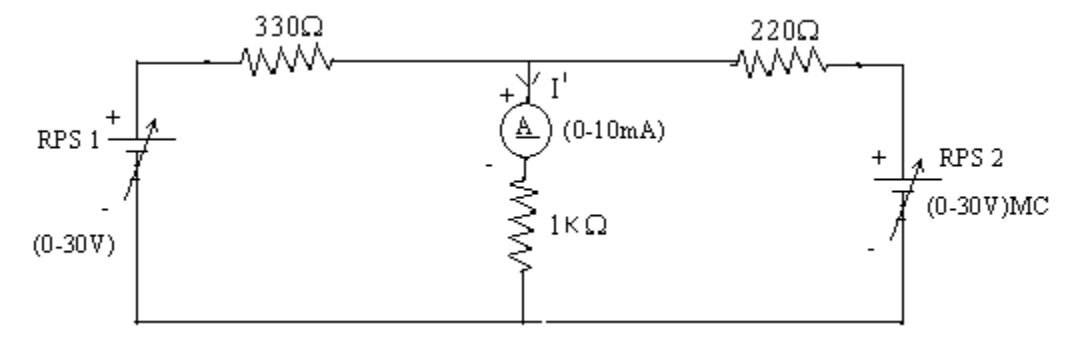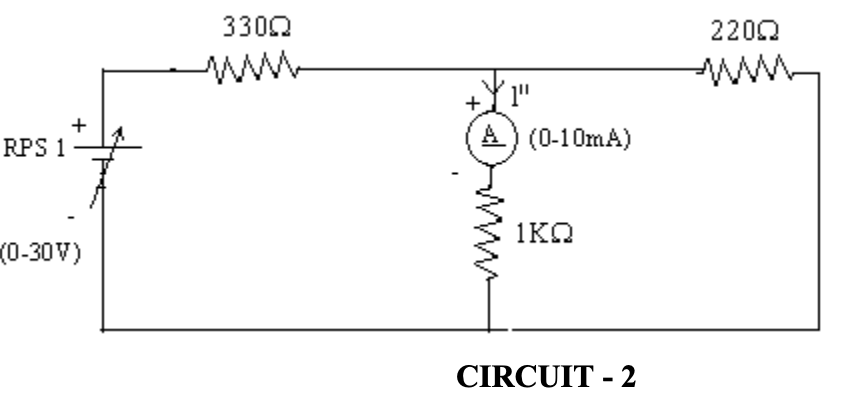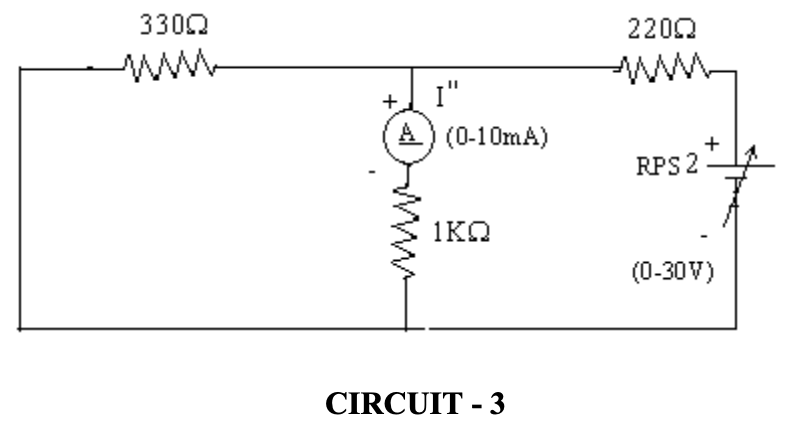# How do you verify superposition theorem?

Superposition theorem states that the response across any element in a linear bilateral network having more than one source is the sum of the responses obtained from each source considered separately while all the other sources are replaced by their internal resistances. Superposition theorem is used to solve networks where two or more sources are present. Superposition Theorem is only applicable to circuits that obey the Ohm’s Law.

### Verification of Superposition Theorem

The superposition theorem can be practically verified using the following experiment.

#### Circuit Diagram#### Procedure

1. Connect the circuit as shown in the figure above.
2. Set RPS1 and RPS2 to a particular voltage as shown in circuit 1 and note down the ammeter reading.
3. Now set the same voltage using RPS1 alone shorting RPS2 and note down the ammeter reading as shown in circuit 2.
4. Set the same voltage using RPS2 shorting RPS1 and note down the ammeter reading as shown in circuit 3.
5. Verify superposition theorem.

#### Verification

Theoretical Values:

 RPS Ammeter Reading 1 2 Circuit 1 10 V 10 V I = 8.83 Circuit 2 10 V 0 V I’= 3.5 Circuit 3 0 V 10 V I”= 5.3

I = I’ + I” = 8.83

Practical Values

 RPS Ammeter Reading 1 2 Circuit 1 10 V 10 V I = 8.5 Circuit 2 10 V 0 V I’= 3.5 Circuit 3 0 V 10 V I”= 5

I = I’ + I” = 8.5 mA = 3.5 + 5 = 8.5 mA

Superposition theorem is therefore practically and theoretically verified.(2)(1)